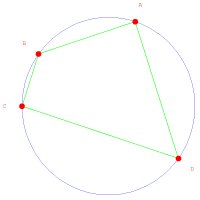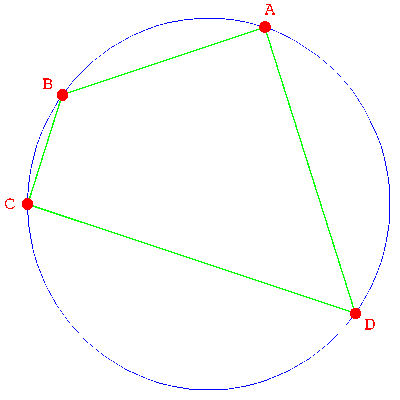Sergey Sadov proved in 2004 the following theorem in elementary geometry.
 THEOREM: If ABCD is a quadrilateral on a circle, then ``` |AB| |BC| |CA| + |AC| |CD| |DA| = |BC| |CD| |DB| + |AB| |BD| |DA| ``` Proof. (This is a Mathematica version of a Maple proof found by Shalosh B. Ekhad, the computer collaborator of Doron Zeilberg at Rutgers university). ``` H[A_,B_]:=(A[]-B[])^2+(A[]-B[])^2; P[t_]:={t+1/t,(t-1/t)/I}/2; T[A_,B_,C_,D_]:=((A+B-C-D)^2-4*(A*B+C*D))^2-64*A*B*C*D; U[A_,B_,C_]:=H[A,B]*H[B,C]*H[C,A]; K={P[t],P[t],P[t],P[t]}; S=T[U[P[t],P[t],P[t]],U[P[t],P[t],P[t]], U[P[t],P[t],P[t]],U[P[t],P[t],P[t]]]==0; Simplify[S] ``` Side remark: Ekhads Maple Proof uses a 360 Character Maple code, which proves it in 0.05 seconds. The above Mathematica code has 336 characters and needed 0.01 seconds to complete on a Pentium D 840.